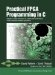# Section 5.2. The FIR Filter Hardware Process

### 5.2. The FIR Filter Hardware Process

The hardware process representing our 51-tap FIR filter is shown in its entirety in Figure 5-1. As with the HelloFPGA example presented in the preceding chapter, this process accepts data on an input stream, filter_in, and performs a repetitive calculation on that stream to produce a corresponding filtered output stream, filter_out.

Stepping through the process, notice the following:

• The only interfaces into and out of the process are via the two streams filter_in and filter_out. An FIR filter such as this requires coefficients in order to perform its operation, so these values (which in the case of this filter are 32-bit integer values) are passed via the same input stream as the data to be filtered, presumably as a part of the system initialization. (A later design refinement might be to obtain the coefficients via a shared memory resource or to modify the process such that new coefficients could be dynamically loaded in response to an input signal.)

• Two local arrays, coef and firbuffer, are defined at the start of the process along with variables nSample, nFiltered, accum, and tap. You can see from the definition of TAPS (which is 51) and the data width (32 bits) that these arrays each equate to 204 bytes (1632 bits) of storage. In traditional C programming you know that such arrays declared within a subroutine are allocated on the stack, and that in all likelihood there is plenty of stack space available for such modestly sized arrays. When targeting hardware, however, you may need to consider (and perhaps specify) where such memory will be allocated and how that allocation relates to the trade-offs of the target platform. For now, however, we'll just take an abstract view of the application, leaving such considerations for a later discussion.

• After the input and output streams are opened (using modes O_RDONLY and O_WRONLY, respectively), the 51 coefficients are read from the input stream filter_in using co_stream_read. The assumption here is that the upstream process (the one feeding inputs to the FIR filter) is well-behaved and will send exactly 51 coefficients on the stream before it starts sending the data to be filtered. If the upstream process has been properly written (the programmer hasn't made an "off by one" or similar error), this method of counting the number of coefficients is quite safe. This is because the self-synchronizing nature of streams (which are FIFO buffers) guarantees that no data will be lost, regardless of whether the upstream and downstream processes are running at vastly different rates (such as when the producer process is running in software and the consuming process is running in hardware).

##### Figure 5-1. A 51-tap FIR filter described using Impulse C.
` #define TAPS 51 void fir(co_stream filter_in, co_stream filter_out) {    int32 coef[TAPS], firbuffer[TAPS];   int32 nSample, nFiltered, accum, tap;   co_stream_open(filter_in, O_RDONLY, INT_TYPE(32));   co_stream_open(filter_out, O_WRONLY, INT_TYPE(32));   // First fill the coef array with the coefficients...   for (tap = 0; tap < TAPS; tap++) {       co_stream_read(filter_in, &nSample, sizeof(int32));      coef[tap] = nSample;   }    // Now fill the firbuffer array with the first n values...   for (tap = 1; tap < TAPS; tap++) {            co_stream_read(filter_in, &nSample, sizeof(int32));           firbuffer[tap-1] = nSample;   }    // Now we have an almost full buffer and can start   // processing the streaming waveform samples.   //   // Each time we process a sample we will "shift" the buffer   // one position and read in a new sample.   //   while ( co_stream_read(filter_in, &nSample, sizeof(int32))                             == co_err_none ) {      firbuffer[TAPS-1] = nSample;     accum = 0;     for (tap = 0; tap < TAPS; tap++) {         accum += firbuffer[tap] * coef[tap];     }      nFiltered = accum >> 2;     co_stream_write(filter_out, &nFiltered, sizeof(int32));     for (tap = 1; tap < TAPS; tap++) {         firbuffer[tap-1] = firbuffer[tap];     }    }    co_stream_close(filter_in);   co_stream_close(filter_out); } `

• After the first loop has completed and the coefficients have been read, a second initialization loop (a loop that is outside of the main processing loop of this process) sets up the filter by filling the primary buffer (firbuffer) with enough values to begin the calculations, which take place in the subsequent main processing loop.

• The main processing loop follows the same pattern we observed in the HelloFPGA example of the preceding chapter, in which the return value of co_stream_read serves as the loop condition. This is the most efficient way to code an Impulse C inner code loop and should be used wherever possible.

• The actual calculations to perform the FIR filtering operation are contained in two inner code loops. The first of these two loops performs the filter calculation for the current value (which is an accumulation of products of the input buffer firbuffer and the corresponding coefficient). The second inner code loop (after a result value is written to the output stream) shifts the array by one in preparation for the next iteration of the main processing loop.

• When the filter process detects an end-of-stream condition (indicating that there are no more filter inputs to process), control drops out of the loop and the two streams (filter_input and filter_output) are closed. In an actual hardware implementation it can be assumed that this will never occur; the process will continue processing values until the system is powered down. (If the stream was closed for some reason, the hardware for the FIR filter would become inactive and useless until the system was restarted.) But for desktop simulation, such cleanup after a set of tests have been run against the process can be useful. In fact, if the streams are never closed, the process might never terminate itself, other processes might not close as a result, and the simulation might not complete as expected.Practical FPGA Programming in C
ISBN: 0131543180
EAN: 2147483647
Year: 2005
Pages: 208

Similar book on Amazon